• 在寻找轮廓之前，要进行阈值化处理或者 Canny 边界检测。  • 查找轮廓的函数会修改原始图像。如果你在找到轮廓之后还想使用原始图像的话，应该将原始图像存储到其他变量中。  • 在 OpenCV
一、什么是轮廓
轮廓可以简单地解释为连接所有连续点（沿着边界），具有相同颜色或强度的曲线。轮廓是形状分析和物体检测和识别的有用工具。

• 为了更加准确，要使用二值化图像。在寻找轮廓之前，要进行阈值化处理或者 Canny 边界检测。

• 查找轮廓的函数会修改原始图像。如果你在找到轮廓之后还想使用原始图像的话，应该将原始图像存储到其他变量中。

• 在 OpenCV 中，查找轮廓就像在黑色背景中超白色物体。你应该记住， 要找的物体应该是白色而背景应该是黑色。

二、如何在一个二值图像中查找轮廓：

import numpy as np
import cv2

imgray = cv2.cvtColor(img,cv2.COLOR_BGR2GRAY)
ret, thresh = cv2.threshold(imgray,127,255,0)
contours, hierachy = cv2.findContours(thresh, cv2.RETR_TREE, cv2.CHAIN_APPROX_SIMPLE)

函数 cv2.ﬁndContours() 有三个参数，第一个是输入图像，第二个是轮廓检索模式，第三个是轮廓近似方法。这个参数如果被设置为 cv2.CHAIN_APPROX_NONE，所有的边界点 都会被存储。但是我们真的需要这么多点吗？例如，当我们找的边界是一条直 线时。你用需要直线上所有的点来表示直线吗？不是的，我们只需要这条直线 的两个端点而已。这就是
cv2.CHAIN_APPROX_SIMPLE 要做的。它会将轮廓上的冗余点都去掉，压缩轮廓，从而节省内存开支。
返回值有三个，第一个是图像，第二个是轮廓，第三个是（轮廓的）层析结构，官方给的也是三个，但是自己实践的情况是两个返回值，没有第一个，也可能是编辑器的问题，目前还没有在别的平台试过，如果试过可能会自己删除这一句话。轮廓（第二个返回值）是一个 Python 列表，其中存储这图像中的所有轮廓。每一个轮廓都是一个 Numpy
数组，包含对象边界点（x，y）的坐标。

三、绘制轮廓

函数 cv2.drawContours() 可以被用来绘制轮廓。它可以根据你提供 的边界点绘制任何形状。它的第一个参数是原始图像，第二个参数是轮廓，一 个 Python 列表。第三个参数是轮廓的索引（在绘制独立轮廓是很有用，当设 置为 -1 时绘制所有轮廓）。接下来的参数是轮廓的颜色和厚度等。

cv2.drawContours(img, contours, -1, (0,255,0), 3) #绘制边缘

cv2.imshow('img',img)
cv2.waitKey()
cv2.destroyAllWindows()
官方给的结果是：第一个图显示使用 cv2.CHAIN_APPROX_NONE 的效果， 一共 734 个点。第二个图是使用 cv2.CHAIN_APPROX_SIMPLE 的结 果，只有 4 个点。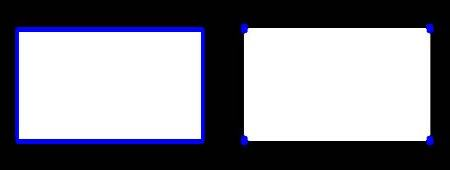实践结果确实只有一种情况： cv2.CHAIN_APPROX_NONE()和cv2.CHAIN_APPROX_SIMPLE()效果一样。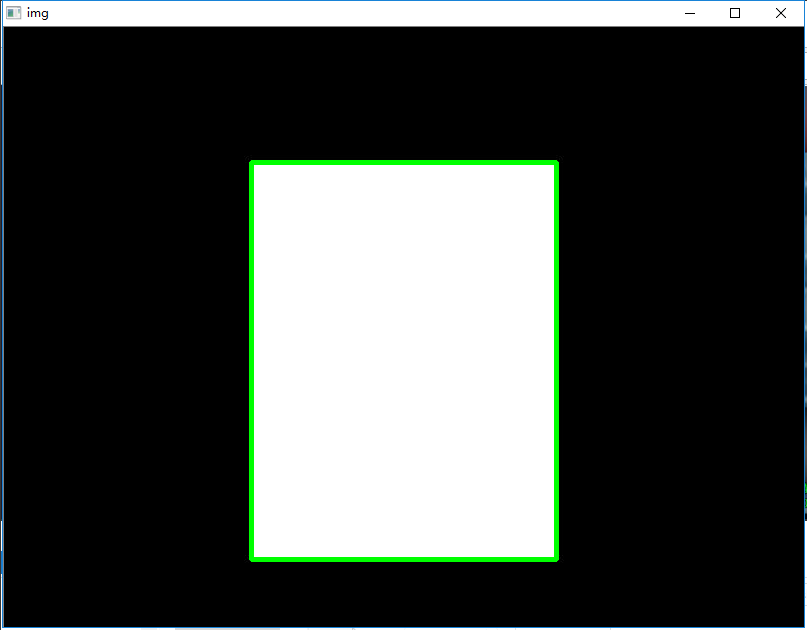拓展：

自己写的程序：

#coding:utf-8
import numpy as np
import cv2

imgray = cv2.cvtColor(img,cv2.COLOR_BGR2GRAY)
ret, thresh = cv2.threshold(imgray,127,255,0)
contours, hierachy = cv2.findContours(thresh, cv2.RETR_TREE, cv2.CHAIN_APPROX_SIMPLE)

for i in range(int(len(contours))): #绘制几个形状的边缘
cnt = contours[i]
cv2.drawContours(img, [cnt], -1, (i*40, 255-i*40, 255-i*40), 3) #用不同的颜色

cv2.imshow('img',img)
cv2.waitKey()
cv2.destroyAllWindows()
效果图：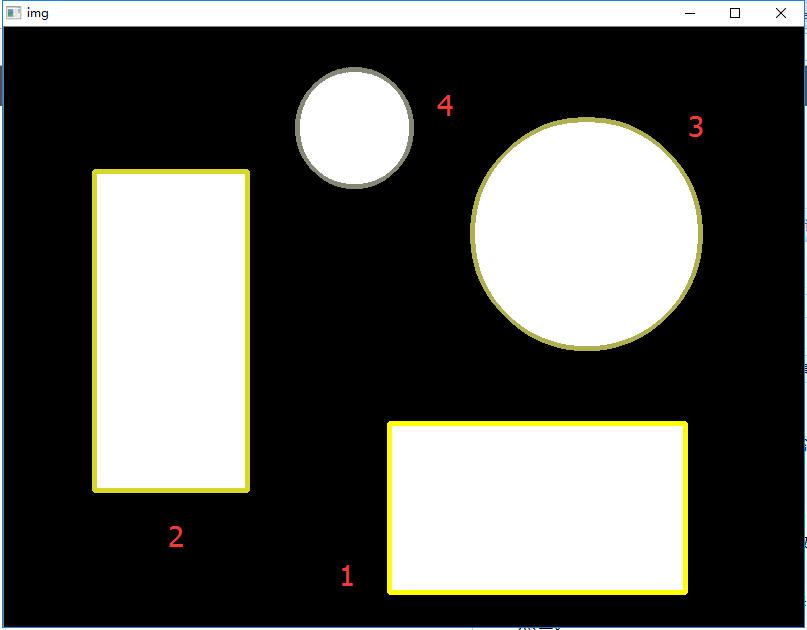展开全文Python
• 学习提取一些常用的物体属性，如坚实度，等效直径，掩模图像，平均强度等。 (注:质心、面积、周长等也属于这一类，但我们在上一章已经见过) 1. 长宽比 它是对象边界矩形的宽度与高度的比值。 Aspect  Ratio=Width...
学习提取一些常用的物体属性，如坚实度，等效直径，掩模图像，平均强度等。
(注:质心、面积、周长等也属于这一类，但我们在上一章已经见过)
1. 长宽比
它是对象边界矩形的宽度与高度的比值。
$Aspect \; Ratio = \frac{Width}{Height}$
x,y,w,h = cv.boundingRect(cnt)
aspect_ratio = float(w)/h

2. 范围
范围是轮廓区域与边界矩形区域的比值。
$Extent = \frac{Object \; Area}{Bounding \; Rectangle \; Area}$
area = cv.contourArea(cnt)
x,y,w,h = cv.boundingRect(cnt)
rect_area = w*h
extent = float(area)/rect_area

3. 坚实度
坚实度是等高线面积与其凸包面积之比。
$Solidity = \frac{Contour \; Area}{Convex \; Hull \; Area}$
area = cv.contourArea(cnt)
hull = cv.convexHull(cnt)
hull_area = cv.contourArea(hull)
solidity = float(area)/hull_area

4. 等效直径
等效直径是面积与轮廓面积相同的圆的直径。
$Equivalent \; Diameter = \sqrt{\frac{4 \times Contour \; Area}{\pi}}$
area = cv.contourArea(cnt)
equi_diameter = np.sqrt(4*area/np.pi)

5. 取向
取向是物体指向的角度。以下方法还给出了主轴和副轴的长度。
(x,y),(MA,ma),angle = cv.fitEllipse(cnt)

6. 掩码和像素点
在某些情况下，我们可能需要构成该对象的所有点。可以按照以下步骤完成：
mask = np.zeros(imgray.shape,np.uint8)

这里提供了两个方法，一个使用Numpy函数，另一个使用OpenCV函数(最后的注释行)。结果也是一样的，只是略有不同。Numpy给出的坐标是(行、列)格式，而OpenCV给出的坐标是(x,y)格式。所以基本上答案是可以互换的。注意，row = x, column = y。
7. 最大值，最小值和它们的位置
我们可以使用掩码图像找到这些参数。
min_val, max_val, min_loc, max_loc = cv.minMaxLoc(imgray,mask = mask)

8. 平均颜色或平均强度
在这里，我们可以找到对象的平均颜色。或者可以是灰度模式下物体的平均强度。我们再次使用相同的掩码进行此操作。
mean_val = cv.mean(im,mask = mask)

9. 极端点
极点是指对象的最顶部，最底部，最右侧和最左侧的点。
leftmost = tuple(cnt[cnt[:,:,0].argmin()])
rightmost = tuple(cnt[cnt[:,:,0].argmax()])
topmost = tuple(cnt[cnt[:,:,1].argmin()])
bottommost = tuple(cnt[cnt[:,:,1].argmax()])

例如，如果我将其应用于印度地图，则会得到以下结果：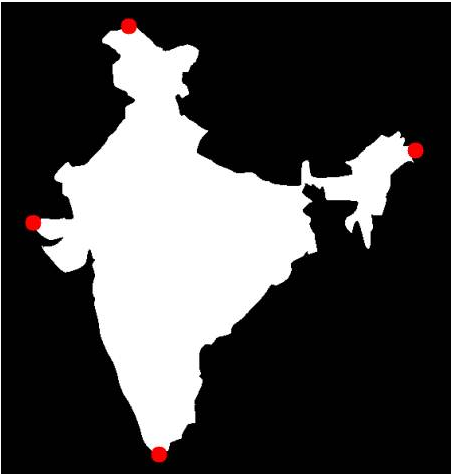展开全文opencv python
• 文章目录OpenCV中的轮廓1.初识轮开阔1.1 原理1.2 常用函数2.轮廓的特征2.1 矩直方图图像变换模板匹配Hough直线变换分水岭算法图像分割使用GrabCut算法进行交互式前景提取 ...寻找轮廓之前要进行阈值化处理或Ca...
OpenCV中的轮廓1.初识轮廓1.1 原理1.2 常用函数2.轮廓的特征2.1 矩2.2 轮廓近似2.3 凸包2.4 边界2.5 拟合2.6 极点3. 形状匹配4. 轮廓的层次结构4.1 轮廓的层级结构4.2 轮廓的检索形式
1.初识轮廓
1.1 原理

轮廓可以简单认为成将连续的点(连着边界)连在一起的曲线，具有相同的颜色或者灰度。

使用二值化图像可以更准确识别轮廓。寻找轮廓之前要进行阈值化处理或Canny边界检测；
查找轮廓会修改原始图像；
OpenCV中查找轮廓类似于在黑色背景中找白色物体。背景是黑色，物体是白色。

1.2 常用函数

查找轮廓：cv2.findContours()。第一个是输入图像，第二个是轮廓检索模式，第三个是轮廓近似方法。
绘制轮廓：cv2.drawContours()。第一个参数是原始图像，第二个参数是轮廓，第三个是轮廓的索引（-1表示绘制所有轮廓）。
轮廓的近似方法：

cv2.CHAIN_APPROX_NONE，存储所有边界点；
cv2.CHAIN_APPROX_SIMPLE，只存储有用的点，去掉冗余点，压缩轮廓。

#!/usr/bin/env python
# -*- coding:utf-8 -*-
import cv2
imgray = cv2.cvtColor(img, cv2.COLOR_BGR2GRAY)
ret, thresh = cv2.threshold(imgray, 127, 255, 0)
# 查找轮廓
contours, hierarchy = cv2.findContours(thresh, cv2.RETR_TREE, cv2.CHAIN_APPROX_SIMPLE)
# 绘制轮廓
img = cv2.drawContours(img, contours, 50, (0, 255, 0), 3)
plt.imshow(img, cmap='gray')
plt.title('Original'), plt.xticks([]), plt.yticks([])

结果：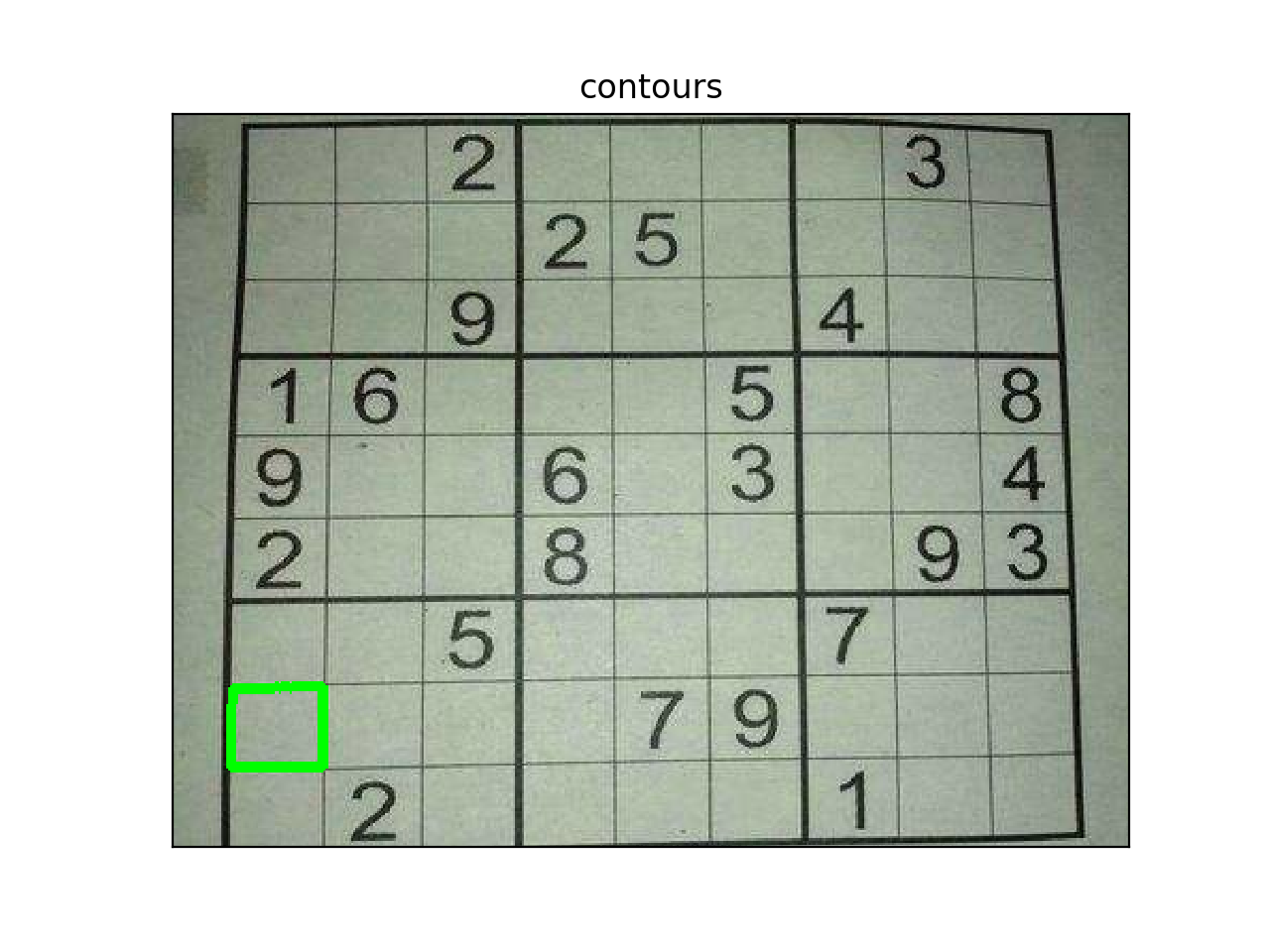2.轮廓的特征
2.1 矩

图像的矩可以用来计算图像的质心，面积等。

#!/usr/bin/env python
# -*- coding:utf-8 -*-
import cv2
ret, thresh = cv2.threshold(imgray, 127, 255, 0)
contours, hierarchy = cv2.findContours(thresh, 1, 2)
cnt = contours
M = cv2.moments(cnt)
print(M)
# 绘制轮廓
# 重心
cx = int(M['m10']/M['m00']) #255
cy = int(M['m01']/M['m00']) #480
#面积‘
area = cv2.contourArea(cnt) #30700
#周长
#  cv2.arcLength()的第二个参数用来指定对象的形状是闭合（True）的还是打开的（一条曲线）。
perimeter = cv2.arcLength(cnt, True) #1162.97

2.2 轮廓近似

将轮廓近似到另一种由更少点组成的轮廓形状。
approx = cv2.approxPolyDP(cnt,epsilon,True)
epsilon：原始轮廓到近似轮廓的最大距离；
True：弧线是否闭合。

# epsilon = 10% 时的近似轮廓
epsilon = 0.1*cv2.arcLength(cnt,True)
approx = cv2.approxPolyDP(cnt,epsilon,True)

2.3 凸包

凸包与轮廓近似相似，但不同，虽然有些情况它们给出的结果是一样的。

cv2.convexHull()：可以用来检测曲线是否具有凸性缺陷，并纠正。
hull = cv2.convexHull(points[, hull[, clockwise[, returnPoints]]])

参数：

points：需要传入的轮廓
hull：输出，通常不需要
clockwise：方向标志。True表示输出的凸包是顺时针方向的，否则逆时针。
returnPoints：默认True，返回凸包上点的坐标；False，返回与凸包点对应的轮廓上的点。

2.4 边界

直边界矩形

一个没有旋转的矩形。不会考虑对象是否旋转，所以得到的边界矩形的面积不是最小的。函数：cv2.boundingRect()。（下图中共绿色框）

旋转边界矩形

因为考虑了对象的旋转，所以这个矩形的面积是最小的。函数：cv2.minAreaRect()。（下图中红色框）

3.最小外接圆

函数cv2.minEnclosingCircle()可以帮我们找到一个对象的最小外切圆。它是能包括对象的圆中面积最小的一个。(下图中蓝色框)

img = cv2.imread("/Users/~/flash.jpg")
# 将图片转化为灰度，再进行二值化
ret, thresh = cv2.threshold(cv2.cvtColor(img.copy(), cv2.COLOR_BGR2GRAY), 127, 255, cv2.THRESH_BINARY)
contours, hier = cv2.findContours(thresh, cv2.RETR_EXTERNAL, cv2.CHAIN_APPROX_SIMPLE)
for c in contours:
# 边界框:
# find bounding box coordinates
# boundingRect()将轮廓转化成(x,y,w,h)的简单边框,cv2.rectangle()画出矩形[绿色(0, 255, 0)]
x, y, w, h = cv2.boundingRect(c)
cv2.rectangle(img, (x, y), (x + w, y + h), (0, 255, 0), 2)

# 最小矩形区域:
# 1 计算出最小矩形区域 2 计算这个的矩形顶点 3 由于计算出来的是浮点数，而像素是整型，所以进行转化 4 绘制轮廓[红色(0, 0, 255)]
# find minimum area
rect = cv2.minAreaRect(c)
# calculate coordinates of the minimum area rectangle
box = cv2.boxPoints(rect)
# normalize coordinates to integers
box = np.int0(box)
# draw contours
cv2.drawContours(img, [box], 0, (0, 0, 255), 3)

# 最小闭圆的轮廓:
# calculate center and radius of minimum enclosing circle[蓝色(255, 0, 0)]
# cast to integers
center = (int(x), int(y))
# draw the circle
img = cv2.circle(img, center, radius, (255, 0, 0), 2)

# 轮廓检测:绘制轮廓
cv2.drawContours(img, contours, -1, (255, 0, 0), 1)
cv2.imshow("contours", img)
cv2.waitKey()
cv2.destroyAllWindows()

结果：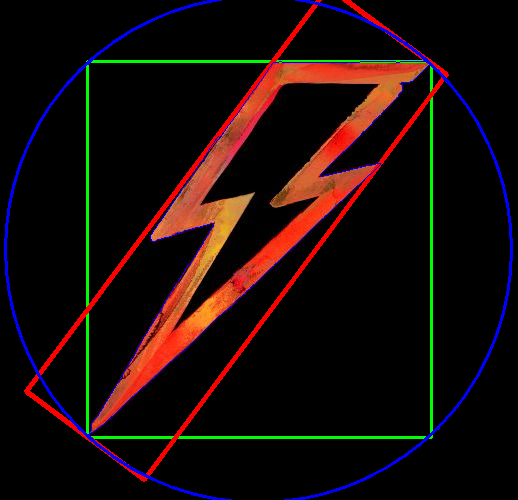2.5 拟合

椭圆拟合

使用函数cv2.ellipse(),返回值其实是旋转边界矩形的内切圆

直线拟合

可以根据一组点拟合出一条直线，同样可以为图中白色点拟合出一条直线

#椭圆拟合，绿色
ellipse = cv2.fitEllipse(c)
img = cv2.ellipse(img, ellipse,(0,255,0),2)

#直线拟合，蓝色线
rows, cols = img.shape[:2]
[vx, vy, x, y] = cv2.fitLine(c, cv2.DIST_L2, 0, 0.01, 0.01)
lefty = int((-x * vy / vx) + y)
righty = int(((cols-x)*vy/vx)+y)
cv2.line(img, (cols - 1, righty), (0, lefty), (255, 0, 0), 2)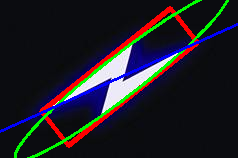2.6 极点
一个图像的最上面、最下面、最左边、最右边的点
    leftmost = tuple(c[c[:,:,0].argmin()])
rightmost = tuple(c[c[:,:,0].argmax()])
topmost = tuple(c[c[:,:,1].argmin()])
bottommost = tuple(c[c[:,:,1].argmax()])

3. 形状匹配
函数cv2.matchShape()可以比较两个形状或轮廓的相似度。如果返回值越小，匹配度越好。
import cv2
import numpy as np

# 二值化
ret, thresh = cv2.threshold(img1, 175, 255, 0)
ret, thresh2 = cv2.threshold(img2, 175, 255, 0)
# 轮廓
contours, hier = cv2.findContours(thresh, 2, 1)
cnt1 = contours
contours, hier = cv2.findContours(thresh2, 2, 1)
cnt2 = contours
#求匹配度
ret = cv2.matchShapes(cnt1, cnt2, 1, 0.0)
print(ret)


![在这里插入图片描述](https://img-blog.csdnimg.cn/201902191904111.jpg)

上左图和中间图的匹配度是3.39
上左图和右图的匹配度是3.99

4. 轮廓的层次结构
4.1 轮廓的层级结构

Next：同一级组织结构中的下一个轮廓，没有的话next = -1；下图中0的Next是1；
Previous：同一级结构中的前一个轮廓，没有的话为-1；1的Previous是0；
First_Chid：便是它的第一个子轮廓，按从上到下，从左到右的顺序排序；没有的话为-1；3a的First_Chid是4；
Parents：表示它的父轮廓，没有的话为-1；5的Parents是3a。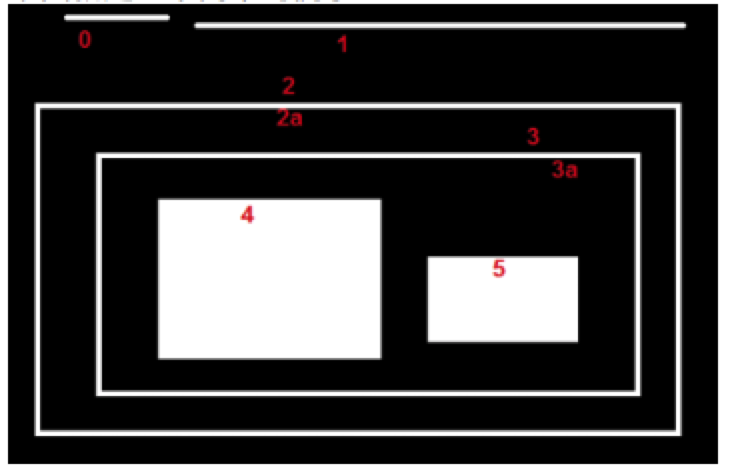4.2 轮廓的检索形式

RETR_LIST：提取所有轮廓，不创建任何父子关系，属于同一级组织轮廓；（不关心轮廓关系的时候用）

RETR_EXTERNAL：返回最外边的轮廓，所有的子轮廓会被忽略；（只想要最外边的轮廓时用）

RETR_CCOMP：返回所有的轮廓，并分为两级组织结构。图中红色字体代表轮廓的编号，绿色代表轮廓的组织层级。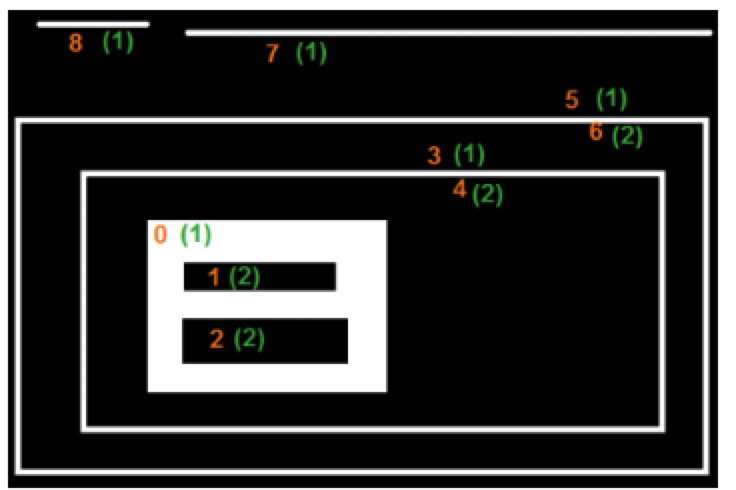结果：
>>> hierarchy
array([[[ 3, -1,  1, -1],
[2,-1,-1, 0],
[-1, 1, -1, 0],
[ 5, 0, 4, -1],
[-1, -1, -1, 3],
[ 7, 3, 6, -1],
[-1, -1, -1, 5],
[8, 5,-1,-1],
[-1, 7, -1, -1]]])


RETR_TREE：最完美的一个，返回所有轮廓，并创建一个完整的组织列表结构。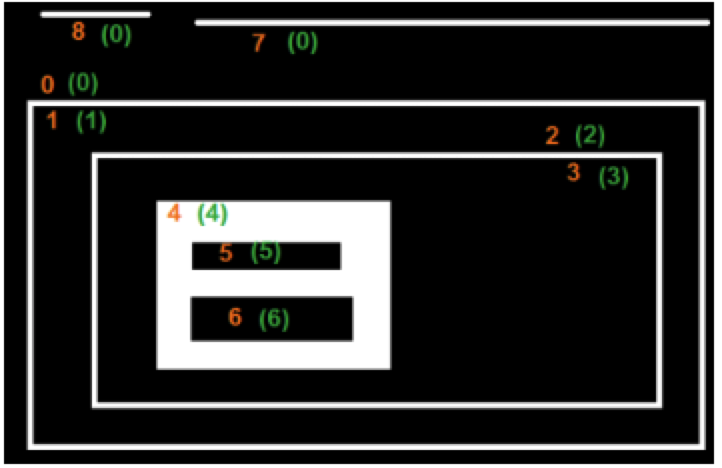结果：

>>> hierarchy
array([[[ 7, -1,  1, -1],
[-1, -1, 2, 0],
[-1, -1, 3, 1],
[-1, -1, 4, 2],
[-1, -1, 5, 3],
[6,-1,-1, 4],
[-1, 5, -1, 4],
[8, 0,-1,-1],
[-1, 7, -1, -1]]])



展开全文• #include "cv.h" #include "highgui.h" using namespace cv; using namespace std; int main(int argc,char *argv[]) { Mat src; src=Mat::zeros(300,300,CV_8UC1); vector vert(6); vert=Point(100,75);... v
使用pointPolygonTest函数获取一个点在轮廓外或轮廓上或轮廓内。
当使用距离测量时，点在轮廓外返回一个负值，越小代表与轮廓距离越远；点在轮廓上时返回0；点在轮廓内将返回一个正值，距离越远值越大。
当不使用距离测量时，负值或正值都是一个恒定值-1或+1

#include "cv.h"
#include "highgui.h"
using namespace cv;
using namespace std;
int main(int argc,char *argv[])
{
Mat src;
src=Mat::zeros(300,300,CV_8UC1);
vector<Point2f> vert(6);
vert=Point(100,75);
vert=Point(200,75);
vert=Point(250,150);
vert=Point(200,225);
vert=Point(100,225);
vert=Point(50,150);
for (int i=0;i<6;i++)
line(src,vert[i],vert[(i+1)%6],Scalar::all(255),1);
imshow("src",src);
vector<vector<Point>> contours;
vector<Vec4i> hierarchy;
findContours(src,contours,hierarchy,RETR_TREE,CHAIN_APPROX_SIMPLE);
Mat raw(src.size(),CV_32FC1);
for (int j=0;j<src.rows;j++)
{
for (int i=0;i<src.cols;i++)
{
raw.at<float>(j,i)=pointPolygonTest(contours,Point2f(i,j),true);//true表示支持距离测量
}
}
double minVal;double maxVal;
minMaxLoc(raw,&minVal,&maxVal);
Mat drawing=Mat::zeros(src.size(),CV_8UC3);
for (int j=0;j<src.rows;j++)
{
for (int i=0;i<src.cols;i++)
{
if (raw.at<float>(j,i)<0)
{
drawing.at<Vec3b>(j,i)=255-int(raw.at<float>(j,i)/minVal*255);
}
else if (raw.at<float>(j,i)>0)
{
drawing.at<Vec3b>(j,i)=255-int(raw.at<float>(j,i)/maxVal*255);
}
else
{
drawing.at<Vec3b>(j,i)=255;
}
}
}
imshow("drawing",drawing);
waitKey(0);
destroyAllWindows();
return 0;
}效果：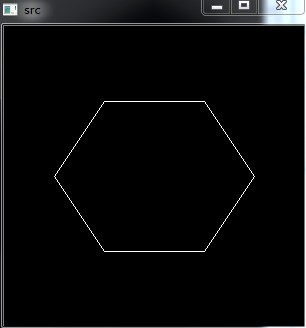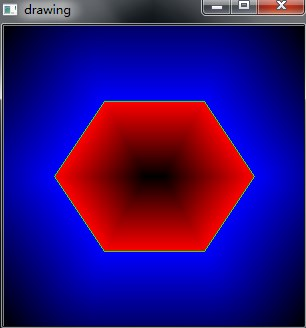不使用距离测量时，结果：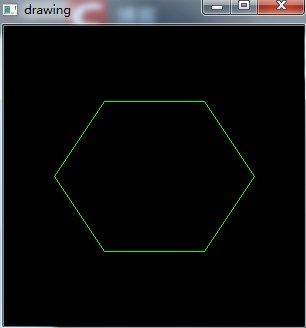展开全文• 好文推荐：http://blog.sina.com.cn/s/blog_891c7ae40101kvtr.html
好文推荐：http://blog.sina.com.cn/s/blog_891c7ae40101kvtr.html
下面展示findContours和drawContours函数

#include "cv.h"
#include "highgui.h"
using namespace cv;
using namespace std;
Mat srcGray;
int lThreshold=100;
void barChange(int pos, void* userdata);
int main(int argc,char *argv[])
{
Mat src;
cvtColor(src,srcGray,CV_BGR2GRAY);
blur(srcGray,srcGray,Size(3,3));
namedWindow("srcGray");
imshow("srcGray",srcGray);
createTrackbar("Low Threshold:","srcGray",&lThreshold,255,barChange);
barChange(0,0);
waitKey(0);
destroyAllWindows();
return 0;
}
void barChange(int pos, void* userdata)
{
Mat srcCanny,dstContours;
vector<vector<Point>> contours;
vector<Vec4i> hierarchy;
Canny(srcGray,srcCanny,lThreshold,lThreshold*2,3);
findContours(srcCanny,contours,hierarchy,CV_RETR_TREE,CHAIN_APPROX_SIMPLE);
cout<<"size:"<<contours.size()<<endl;
dstContours=Mat::zeros(srcCanny.size(),CV_8UC3);
for (int i=0;i<contours.size();i++)
drawContours(dstContours,contours,i,Scalar(0,255,255),1,8,hierarchy,0);
imshow("dstContours",dstContours);
}
实验效果：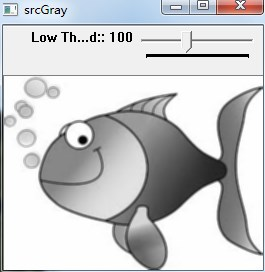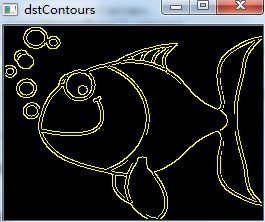轮廓凸包：convexHull

#include "cv.h"
#include "highgui.h"
using namespace cv;
using namespace std;
Mat srcGray;
int lThreshold=100;
void barChange(int pos, void* userdata);
int main(int argc,char *argv[])
{
Mat src;
cvtColor(src,srcGray,CV_BGR2GRAY);
blur(srcGray,srcGray,Size(3,3));
namedWindow("srcGray");
imshow("srcGray",srcGray);
createTrackbar("Low Threshold:","srcGray",&lThreshold,255,barChange);
barChange(0,0);
waitKey(0);
destroyAllWindows();
return 0;
}
void barChange(int pos, void* userdata)
{
Mat srcCanny,dstContours;
vector<vector<Point>> contours;
vector<Vec4i> hierarchy;
Canny(srcGray,srcCanny,lThreshold,lThreshold*2,3);
findContours(srcCanny,contours,hierarchy,CV_RETR_TREE,CHAIN_APPROX_SIMPLE);
cout<<"size:"<<contours.size()<<endl;
vector<vector<Point>> hull(contours.size());
for (int i=0;i<contours.size();i++)
{
convexHull(contours[i],hull[i]);
}
dstContours=Mat::zeros(srcCanny.size(),CV_8UC3);
for (int i=0;i<contours.size();i++)
{
drawContours(dstContours,contours,i,Scalar(0,255,255),2,8,vector<Vec4i>(),0);
drawContours(dstContours,hull,i,Scalar(0,0,255),1,8,vector<Vec4i>(),0);
}
imshow("dstContours",dstContours);
}
效果：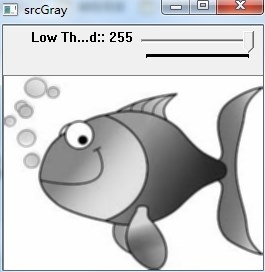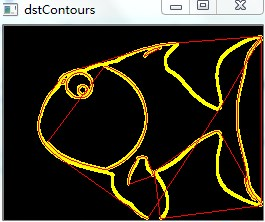创建轮廓矩形框和圆形框
• Use the OpenCV function boundingRect
• Use the OpenCV function minEnclosingCircle
#include "cv.h"
#include "highgui.h"
using namespace cv;
using namespace std;
Mat srcGray;
int lThreshold=100;
void barChange(int pos, void* userdata);
int main(int argc,char *argv[])
{
Mat src;
cvtColor(src,srcGray,CV_BGR2GRAY);
blur(srcGray,srcGray,Size(3,3));
namedWindow("srcGray");
imshow("srcGray",srcGray);
createTrackbar("Low Threshold:","srcGray",&lThreshold,255,barChange);
barChange(0,0);
waitKey(0);
destroyAllWindows();
return 0;
}
void barChange(int pos, void* userdata)
{
Mat srcThreshold,dstContours;
vector<vector<Point>> contours;
vector<Vec4i> hierarchy;
threshold(srcGray,srcThreshold,lThreshold,255,THRESH_BINARY);
findContours(srcThreshold,contours,hierarchy,CV_RETR_TREE,CHAIN_APPROX_SIMPLE);
cout<<"size:"<<contours.size()<<endl;
vector<vector<Point>> poly(contours.size());
vector<Rect> boundRect(contours.size());
vector<Point2f> center(contours.size());

for (int i=0;i<contours.size();i++)
{
approxPolyDP(contours[i],poly[i],3,true);
boundRect[i]=boundingRect(poly[i]);
}
dstContours=Mat::zeros(srcGray.size(),CV_8UC3);
for (int i=0;i<contours.size();i++)
{
drawContours(dstContours,poly,i,Scalar(0,255,255),2,8,vector<Vec4i>(),0);
rectangle(dstContours,boundRect[i].tl(),boundRect[i].br(),Scalar(128,128,128));
}
imshow("dstContours",dstContours);
}<strong style="color: rgb(204, 0, 0); ">
</strong>效果：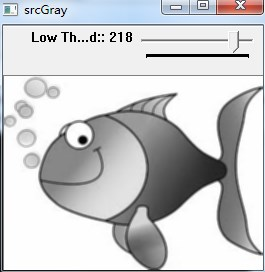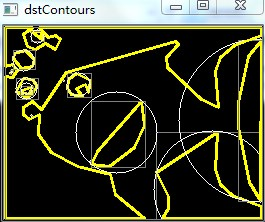#include "cv.h"
#include "highgui.h"
using namespace cv;
using namespace std;
Mat srcGray;
int lThreshold=100;
void barChange(int pos, void* userdata);
int main(int argc,char *argv[])
{
Mat src;
cvtColor(src,srcGray,CV_BGR2GRAY);
blur(srcGray,srcGray,Size(3,3));
namedWindow("srcGray");
imshow("srcGray",srcGray);
createTrackbar("Low Threshold:","srcGray",&lThreshold,255,barChange);
barChange(0,0);
waitKey(0);
destroyAllWindows();
return 0;
}
void barChange(int pos, void* userdata)
{
Mat srcThreshold,dstContours;
vector<vector<Point>> contours;
vector<Vec4i> hierarchy;
threshold(srcGray,srcThreshold,lThreshold,255,THRESH_BINARY);
findContours(srcThreshold,contours,hierarchy,CV_RETR_TREE,CHAIN_APPROX_SIMPLE);
cout<<"size:"<<contours.size()<<endl;

vector<RotatedRect> minRect(contours.size());
vector<RotatedRect> minEllipse(contours.size());

for (int i=0;i<contours.size();i++)
{
minRect[i]=minAreaRect(contours[i]);
if (contours[i].size()>5)
{
minEllipse[i]=fitEllipse(contours[i]);
}
}
dstContours=Mat::zeros(srcGray.size(),CV_8UC3);
Point2f rectPoint;
for (int i=0;i<contours.size();i++)
{
drawContours(dstContours,contours,i,Scalar(0,255,255),2,8,vector<Vec4i>(),0);
ellipse(dstContours,minEllipse[i],Scalar(255,255,255));
minRect[i].points(rectPoint);
for (int j=0;j<4;j++)
{
line(dstContours,rectPoint[j],rectPoint[(j+1)%4],Scalar(180,180,180));
}
}
imshow("dstContours",dstContours);
}
效果：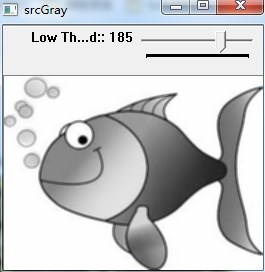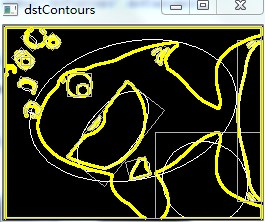展开全文• OpenCV-Python Tutorials，包括对原文档种错误代码的纠正该章节分为以下四个小节：(一) Contours：Getting Started（轮廓：开始）(二) Contours Features（轮廓特征）(三) Contours Properties（轮廓属性）(四) ...python opencv
• 本文内容参考《数字图像处理基础》Wilhelm Burger等著。 根据图像数组获得边缘检测信息，然后循着已检测到的边缘点找到轮廓线。轮廓跟踪：从那些边缘强度较大的地方开始，沿着两个不同方向跟踪边缘点，直到这两条...图像处理
• 实验三 图像轮廓提取与边缘检测 一、实验目的： 理解并掌握对二值图像进行轮廓提取的相关算法（比如，掏空内部点法），以及用于图像边缘检测和提取的典型微分算子（梯度算子和拉普拉斯算子）。 二、实验环境...图像处理 数字
• 图像轮廓检测 （一）检测轮廓 在OpenCV-python中，使用cv2.findContours()函数来对图像进行轮廓检测。 返回三个值：image，contours，hierarchy contours指的是轮廓本身，hierarchy是每条轮廓对应的属性 cv2....图像轮廓检测
•  二值图像轮廓提取对于图像识别，图像分割有着重要意义。该算法的核心就是将图像目标的内部点消除。所谓内部点，我们要根据当前像素点的邻域来进行判断，假设邻域窗口为3*3窗口，如果当前像素P(x,y)的八个邻域...
• 什么是图像轮廓？在这里不去深入研究它的定义，简单的理解，当人站在太阳下，产生的影子的边界就是轮廓。大致是这样子吧。 #轮廓提取的原理做为一个初学者，试想如果提取轮廓？我首先想到的是沿着轮廓边界搜索。很...
• 关于“轮廓检测”和“边缘检测”这两个自己也弄的不是特别清楚，可能确实比较相似吧。下面简单说一下自己的看法。 区别： 边缘检测主要是通过一些手段检测数字图像中明暗变化剧烈（即梯度变化比较大）像素点，偏向...
• 数字图像处理-图像分割：Snake主动轮廓模型 Matlab代码及运行结果， 提供了详细的代码实现和相关说明，会对需要的人提供帮助。程序已在Matlab2012b上测试通过，有疑问请留言图像处理
• 上学那会儿，我们同学说“学这微积分有什么啊，我去买菜还积个分呢？”N年之后终于用上了。轮廓匹配
• 快乐虾...测试图像仍然是它：首先要做的当然是对图像进行分区域处理。在上一步中我们得到了标识绿色植物的二值图像，一个很自然的想法是利用此二值图像轮廓进行分块。[python] view plain copy ...python opencv image
• 图像轮廓凹陷修补 <br /> 轮廓凸外形，可以认为是一种不带限制条件的简单凹陷修补。考虑更为普遍的情况，目标对象总体上呈凸外形，而局部存在轻微凹陷。对于这类目标对象，如果存在 非常严重的凹陷，通常是...null float
• 实验目的：本代码主要是对一幅灰度图像rice.jpg进行一些处理，消除rice.jpg图像中的亮度不一致的背景，并使用阈值分割将修改后的图像转换为二值图像，使用轮廓检测返回图像中目标对象的个数以及统计属性。...图像处理 object matlab
•图像形态学
• 图像轮廓缺陷修补 <br />  通过简单的二值化和边缘提取可以得到封闭的图像轮廓。但大多数边缘提取算子得到的轮廓都可能存在缺陷，即轮廓不封闭。...图像轮廓修补仅需要对轮廓端点进行处理，而float
• 如题，由于图像轮廓具有大量的数据点，我是用的opencv的findcontours提取的数据点，但是我不知道它的数据点的顺序，那该怎么进行曲线拟合呢？opencv 图像处理 数据 曲线拟合
...